Technical Article

# What Are the DNL and INL Specifications of a DAC? Non-Linearity in Digital-to-Analog Converters

March 13, 2019 by Dr. Steve Arar

## This article looks at the DNL and INL specifications of a digital-to-analog converter (DAC).

This article looks at the DNL and INL specifications of a digital-to-analog converter (DAC).

We can use several different specifications to characterize the performance of a data converter. Depending on the application, some of these specifications might be more important than the other ones.

This article looks at the DNL (differential nonlinearity) and INL (integral nonlinearity) specifications of a DAC. These specs are generally important in measurement and control applications where there is usually an error budget from an ideal transfer characteristic.

### Introduction to Output Voltage in a Three-Bit Unipolar DAC

A digital-to-analog converter (DAC) receives data represented as a digital code and produces an equivalent analog output (See Figure 1 below).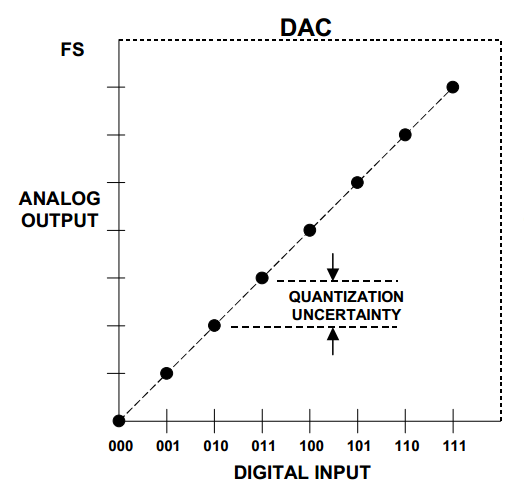##### Figure 1. Image courtesy of Analog Devices.

The above ideal transfer function corresponds to a three-bit unipolar DAC. Various structures, such as a Kelvin Divider or an R-2R architecture, can be used to obtain the above input-output characteristic.

For the purpose of illustrating the DNL and INL specifications, we’ll consider a simple current source–based structure, as shown in Figure 2.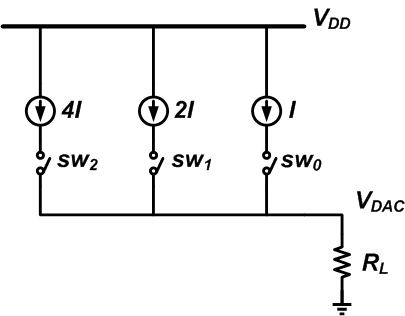##### Figure 2

This three-bit DAC uses binary-weighted current sources to produce the eight different analog levels of Figure 1. Here, I is the unit current that can produce the smallest voltage step in the DAC output. Hence, sw0 and sw2 correspond respectively to the least significant bit (LSb) and most significant bit (MSb) of the input code.

As an example, if the input digital code is 101, the switches sw0 and sw2 are turned on and the load current is I + 4I = 5I. This produces an output voltage equal to 5I ✖ RL. Similarly, other combinations of the input digital code will produce one of the eight different levels of Figure 1.

### How to Characterize Non-Ideal Output Voltage Levels

The above model of a three-bit DAC in Figure 2 is too idealistic; in reality, there are many factors that limit the accuracy of the system. For example, although we want a binary-weighted set of current sources, the values of the current sources may be slightly different from those of Figure 2.

An exaggerated case is shown in Figure 3.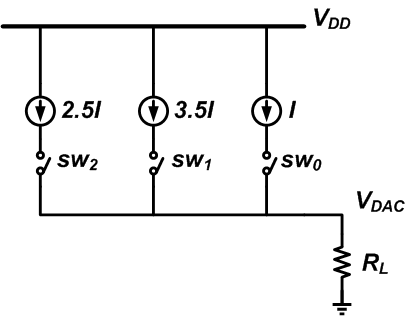##### Figure 3

The input-output characteristic of this non-ideal DAC is shown in Figure 4. Ideally, the output voltage levels should be on the dashed straight line; however, due to the mismatch, we get the voltage levels denoted by the solid points.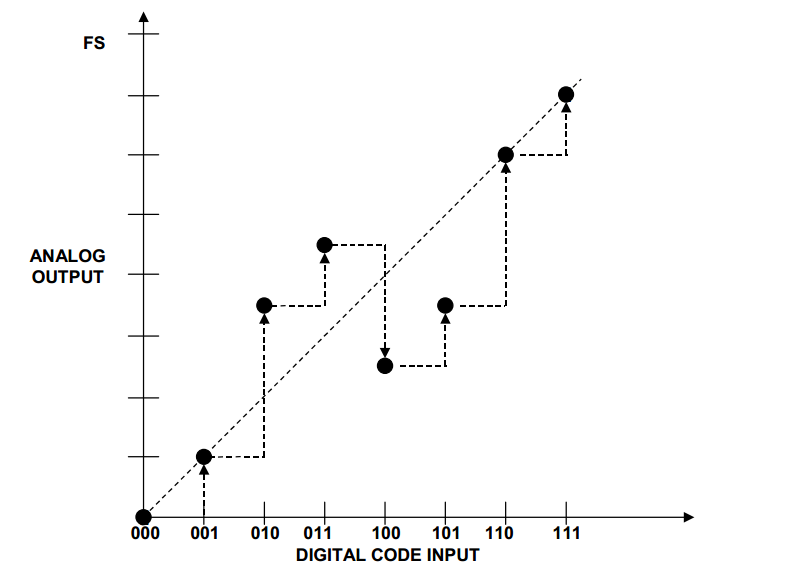##### Figure 4

The DNL and INL specifications of a DAC allow us to characterize such non-ideal output voltage levels.

### Differential Non-Linearity (DNL)

With the ideal transfer characteristic, if we increase the input digital code by one, the analog output should increase by the analog value corresponding to one LSb.

For example, if the input code of Figure 2 changes from 001 to 010, the output should go from I ✖ RL to 2I ✖ RL. The difference between these two levels is I ✖ RL, that is, the analog value of one LSb. However, if we change the digital code of Figure 4 from 001 to 010, the output will change from I ✖ RL to 3.5I ✖ RL. In this case, the difference between the produced levels is 2.5I ✖ RL.

The DNL specification is a way to characterize the difference between two successive voltage levels that a DAC produces. The DNL is the maximum deviation of the output steps from the ideal analog LSb value.

#### How to Calculate DNL

For example, considering the transition from 001 to 010, we get an output step of 2.5I ✖ RL. The deviation of this step from one LSb value is 2.5I ✖ RL - I ✖ RL = 1.5I ✖ RL. Hence, the DNL of this transition is 1.5 LSb. The DNL of the other transitions is shown in Figure 5.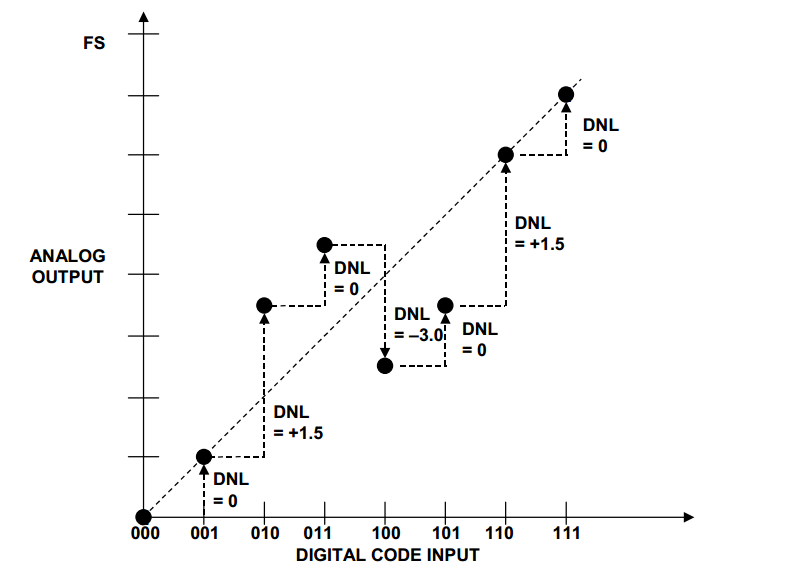##### Figure 5. Image courtesy of Analog Devices

As you can see, the DNL of a non-ideal DAC can be positive, negative, or zero for the different transitions. We usually use the DNL of the largest deviation for all the transitions to specify the DAC DNL performance. For example, the worst DNL error for the input-output characteristic of Figure 5 is -3 LSb.

The DNL is usually measured in LSb, although we can measure it as an absolute value (either volts or amperes) or as a percentage of the full-scale value.

### Integral Non-Linearity (INL)

The INL is defined as the maximum deviation of the actual input-output characteristic from the ideal transfer characteristic. The ideal transfer characteristic is a straight line going through the origin and the point corresponding to the all 1’s digital code (full scale value minus one LSb). This line is called the endpoint-fit line in some textbooks, and this method of measuring the INL is called the endpoint method.

With the example of Figure 5, the INL of the output for code 100 is -1.5 LSb (the ideal output should be 4 LSb but the actual output is 2.5 LSb).

It’s worthwhile to mention that another definition of INL calculates the maximum deviation from an ideal interpolating line. This method is sometimes referred to as the “best straight line method”. The endpoint-fit method is usually preferred because it gives us more information about harmonic distortion of the DAC. Hence, we’ll use the endpoint-fit definition in this article.

#### How to Calculate INL

The INL for the output of any code can be found by calculating the algebraic sum of all the DNLs of the previous transitions. Hence, we have the following relation:

$$INL_{n} = \sum_{i=0}^{n} DNL_{i}$$

For example, the INL for the output of code 100 is equal to 0 + (+1.5) + 0 + (-3) = -1.5 LSb. Since INL characterizes the deviation from the ideal transfer curve, it’s kind of analogous to the linearity error of an amplifier. Just like the DNL, the INL is usually measured in LSb, although we can measure it as an absolute value (either volts or amperes) or as a percentage of the full scale value.

In the rest of this article, we’ll explore the relationship between the DNL and INL metrics. We’ll consider a simple unary-weighted current source–based structure (shown in Figure 6) to discuss the concepts.

### Unary Weighted Current Source–Based DAC

A binary-weighted current source–based DAC is shown in Figure 2. Instead of using binary-weighted current sources, we can use current sources of the same value to implement a DAC. This method, which uses a unary-weighted current source–based structure, is shown in Figure 6.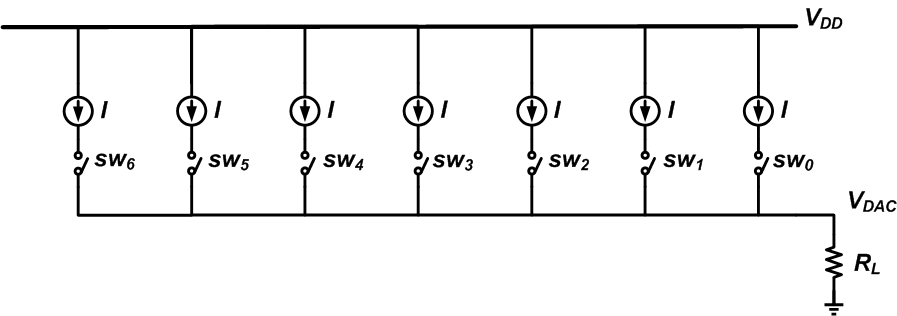##### Figure 6

In this case, an appropriate number of switches should be turned on to produce a given analog voltage level. For example, if the input digital code is 101, we can turn on the first five switches (sw0 to sw4) and produce a load current of 5I. Note that a digital circuit is required to convert the binary DAC input code to the appropriate code that turns on the switches.

As the binary input code increases, we can turn on the current sources in any order. However, the simplest way is to turn on the current sources in order from, say, right to left. In other words, as the digital input code increases, switches sw0, sw1, …, sw6 are turned on in order.

### Random Errors and Systematic Errors

Just like the case of the binary-weighted version, the current sources of the unary-weighted DAC can exhibit deviation from the ideal value. The array of current sources may experience two types of errors: random errors and systematic errors. To gain a better understanding of these errors, assume that a single PMOS transistor is used to implement each of the current sources of Figure 6. This is shown in Figure 7.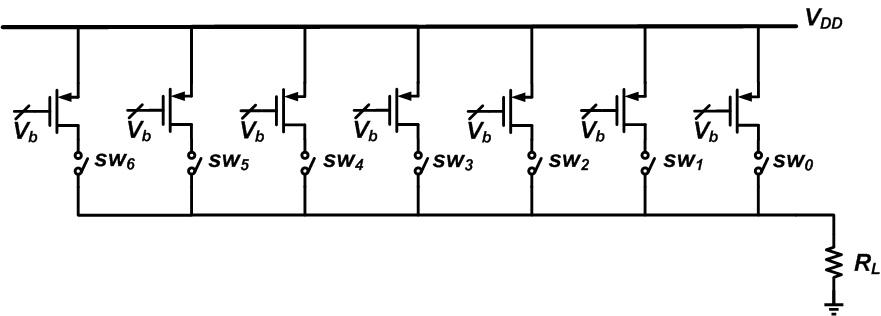##### Figure 7

In this figure, Vb represents the bias voltage, and the transistors have identical dimensions. Although identical current sources are desired, we’ll have slightly different currents. This is due to the fact that many fabrication parameters are in fact random variables. For example, the edge of different layers used in an IC fabrication process exhibits random variations. Or, the diffusion of impurities involves randomness across the wafer. Due to these random variations, we expect mismatch between the transistor currents.

In addition to these random variations, there can be systematic errors too.

An example of these systematic errors is shown in Figure 8.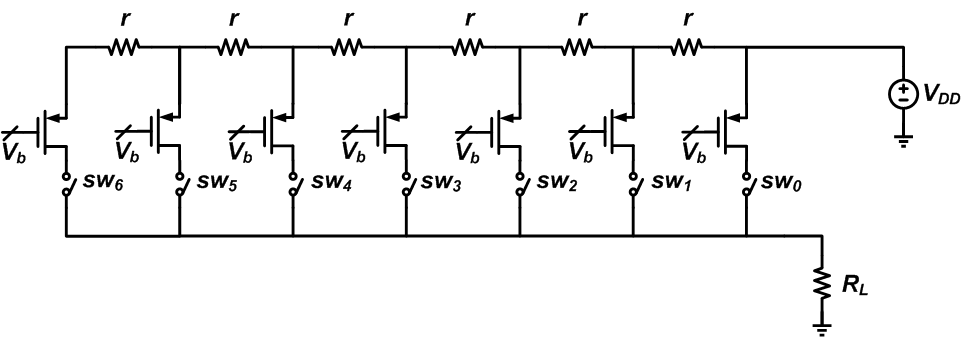##### Figure 8

This figure includes the resistance of the wire that connects VDD to the transistors. Each of the transistors sees a different resistance and consequently a different voltage drop from VDD. As a result, even if the transistors are 100% identical, their current will be slightly different. Note that this is not a random error.

### DNL: The Correlated and Uncorrelated Terms

Considering the discussion of the previous section, we can decompose the DNL error of each DAC transition into two parts: the correlated term (єcorr, caused by the systematic errors) and the uncorrelated term (єuncorr, caused by the random variations). Hence, we have

$$DNL_{i} = \epsilon_{corr} + \epsilon_{uncorr}$$

We know that the INL of a particular output level is the running sum of the DNL of all the previous DAC transitions. The sum of the uncorrelated terms of the DNL will look like a noise term and will increase the noise floor of the DAC output spectrum (we’ll have reduced signal-to-noise ratio). The sum of the correlated terms can build up and contribute to a considerable INL value. A large INL will lead to harmonic distortion (in the DAC output spectrum, we’ll have harmonic components rather than increased noise floor).

As you can see, a large DNL is not necessarily accompanied by a large INL, because if єuncorr is much larger than єcorr, we shouldn’t have a large INL. In this case, the large DNL can be viewed as a source of extra noise.

I mentioned above that we can turn on/off the current sources of Figure 6 in order from right to left (as the digital input code increases, switches sw0, sw1, …, sw6 are turned on in order). There are techniques for dynamically changing the order of switching the current sources on/off. Using such techniques, we can achieve a pseudo-random order and thus decrease the systematic components of the DNL error. This can effectively improve the DAC linearity.

In this article, we looked at the DNL and INL specifications of a DAC. These specs are often important in measurement and control applications. We saw that the DNL error can be decomposed into two parts: the correlated term (єcorr) and the uncorrelated term (єuncorr). The uncorrelated part usually reduces the signal-to-noise ratio, and the correlated part leads to harmonic components.•Ashenafi Kebede June 19, 2019

Like.
• R
r7aaex August 23, 2019

I like Figure 5 and the example that you give.  An improvement to the example might be the DNL calculation that you graphed.  It would go like this, assume that RL = 1 and that current I =1.  The data table you have to generate Fig5 is:
Decimal Code   Vout
0                   0
1                   1
2                   3.5
3                   4.5
4                   2.5
5                   3.5
6                   6
7                   7

To generate DNL take the difference of delta Vout from one transition to the next and ideal value.  Our table might look like this:

Decimal Code   Vout   DNL
0                   0
1                   1       (1-0) - 1   = 0
2                   3.5     (3.5-1) - 1 =1.5
3                   4.5     (4.5-3.5) - 1 = 0
4                   2.5     (2.5-4.5) - 1 = -3
5                   3.5     (3.5 - 2.5)-1 = 0
6                   6       (6 - 3.5) - 1 =1.5
7                   7       (7 - 6) - 1   = 0

These numbers match the numbers in the graph.

Like.